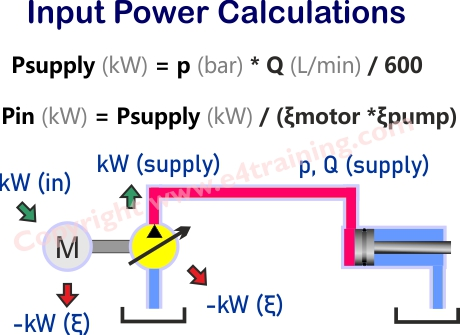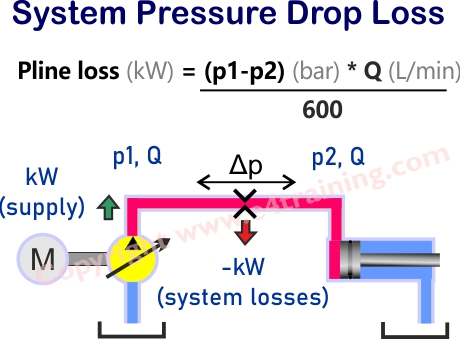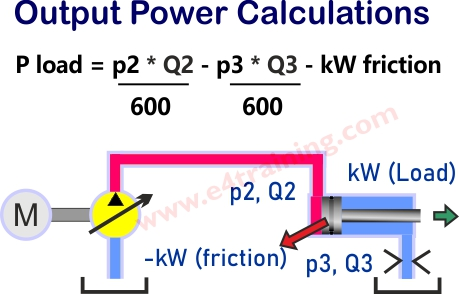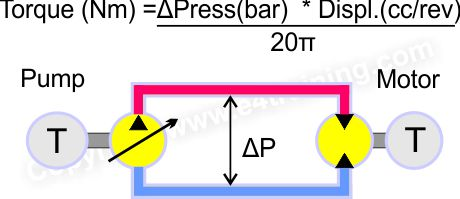# Fluid Power Fundamentals

## Basic power and torque formulas and hydraulic calculations

Understand the power and torque principles.
Self-study lesson plans and training record download page.

### Hydraulic power generated by the prime moverThe INPUT POWER required by an electric motor can be calculated from the OUTPUT POWER provided by the pump, less the INEFFICIENCIES of the pump and motor, or power supply system (prime mover).

Pin = p * Q /(600 * Eff.pump * Eff.motor)

There will also be significant differences between the mechanical and volumetric efficiency of different pump designs, especially when looking at them across their speed, pressure and temperature ranges. It's not unusual for designers to simply add an extra 15% safety margin to remove the risk of failures, particularly as it can be difficult to get hold of manufacturer's efficiency figures across all operating conditions.

### Pipeline and valve power lossesThe pressure at the actuator P2 equals the pressure from the supply P1, less the line and valve losses. In practice, you may also need to consider the losses between the actuator and fluid reservoir as well, although these are likely to be less significant.

The energy lost within the pipework, and pump or actuator inefficiencies, will all be converted into heat. By calculating the total power loss in the system we have a pretty accurate figure for the total heat generated by the system.

### Hydraulic power at the actuatorThe POWER available at the ACTUATOR is equal to the PRESSURE P2 multiplied by the FLOW. However, if the actuator return line pressure is high then the PQ power used there must be deducted from the available power.

The power output from the Actuator will be equal to the power into the actuator less the actuator losses and the return side power used to control the actuator movement. The actual power, or work done by, the actuator can only be calculated from the mechanical loads and these will be different on every system.

See also the advanced training for calculations for accumulators, pressure compensation, stiffness, cooling etc.

### Hydraulic Torque CalculationsThe conversion of hydrostatic pressure into torque or torque back into hydrostatic pressure is the same for all hydraulic pumps and motors. If we ignore inefficiencies we can use the basic formula TORQUE = PRESSURE * SWEPT VOLUME / 2*PI

T (Nm) = Press(bar) * Disp (cc/rev) / 20*PI = XX Nm

For example, if our 10cc/rev pump or motor sees 100 bar then the torque required to drive the pump or exerted by the motor output shaft will be approximately T (Nm) = 100(bar) * 10 (cc/rev) / 20*PI = 16 Nm

But don't forget to take into account any gearbox or linkage ratios and using imperial units will be different.